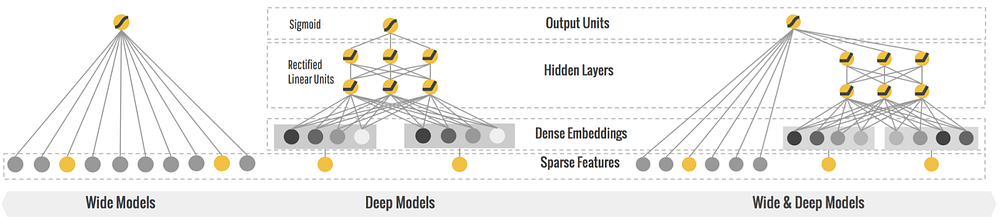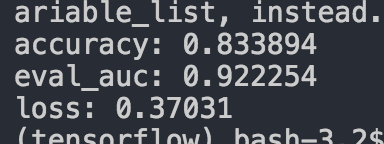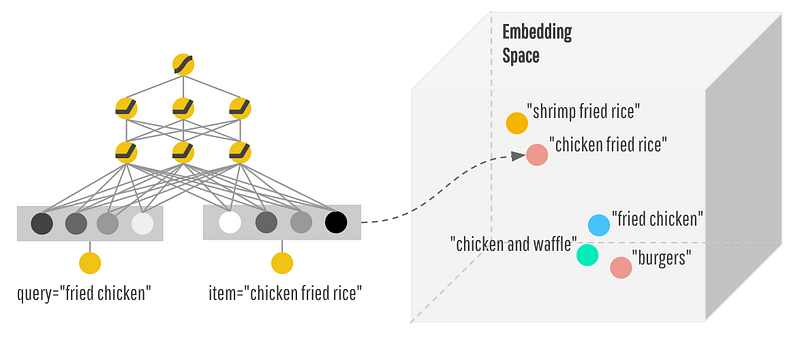TensorFlow in a Nutshell — Part Two: Hybrid Learning

The fast and easy guide to the most popular Deep Learning framework in the world.

Make sure to check out Part One: Basics

In this article we will Demonstrate a Wide ‘N Deep Network that will use wide linear model trained simultaneously with a feed forward network for more accurate predictions than some tradition machine learning techniques. This hybrid learning method will be used to predict Survival probability of Titanic passengers.

These hybrid learning methods are already in production by Google in the Play store for app suggestions. Even Youtube is using similar hybrid learning techniques to suggest videos.

Wide and Deep Network

A Wide and Deep Network combines a linear model with a feed forward neural net so that our predictions will have memorization and generalization. This type of model can be used for classification and regression problems. This allows for less feature engineering with relatively accurate predictions. Thus, getting the best of both worlds.The Data

We are going to be using the Titanic Kaggle data to predict whether or not the passenger will survive based on certain attributes like Name, Sex, what ticket they had, the fare they paid the cabin they stayed in etc. For more information on this data set check out here at Kaggle.

First off we’re going to define all of our columns as Continuos or Categorical.

Continuous columns — any numerical value in a continuous range. Pretty much if it is a numerical representation like money, or age.

Categorical columns — part of a finite set. Like male or female, or even what country someone is from.

CATEGORICAL_COLUMNS = ["Name", "Sex", "Embarked", "Cabin"]
CONTINUOUS_COLUMNS = ["Age", "SibSp", "Parch", "Fare", "PassengerId", "Pclass"]

Since we are only looking to see if a person survived, this is a binary classification problem. We predict a 1 if that person survives and a 0… if they do not :( , We then create a column solely for our survived category.

SURVIVED_COLUMN = "Survived"

The Network

Now we can get to creating the columns and adding embedding layers. When we build our model were going to want to change our categorical columns into a sparse column. For our columns with a small set of categories such as Sex or Embarked (S, Q, or C) we will transform them into sparse columns with keys

sex = tf.contrib.layers.sparse_column_with_keys(column_name="Sex",
keys=["female",
"male"])
embarked = tf.contrib.layers.sparse_column_with_keys(column_name="Embarked",
keys=["C",
"S",
"Q"])

The other categorical columns have many more options than we want to put keys, and since we don’t have a vocab file to map all of the possible categories into an integer we will hash them.

cabin = tf.contrib.layers.sparse_column_with_hash_bucket(
"Cabin", hash_bucket_size=1000)
name = tf.contrib.layers.sparse_column_with_hash_bucket(
"Name", hash_bucket_size=1000)

Our continuous columns we want to use their real value. The reason passengerId is in continuous and not categorical is because they’re not in string format and they’re already an integer ID.

age = tf.contrib.layers.real_valued_column("Age")
passenger_id = tf.contrib.layers.real_valued_column("PassengerId")
sib_sp = tf.contrib.layers.real_valued_column("SibSp")
parch = tf.contrib.layers.real_valued_column("Parch")
fare = tf.contrib.layers.real_valued_column("Fare")
p_class = tf.contrib.layers.real_valued_column("Pclass")

We are going to bucket the ages. Bucketization allows us to find the survival correlation by certain age groups and not by all the ages as a whole, thus increasing our accuracy.

age_buckets = tf.contrib.layers.bucketized_column(age,
boundaries=[
5, 18, 25,
30, 35, 40,
45, 50, 55,
65
])

Almost done, we are going to define our wide columns and our deep columns. Our wide columns are going to effectively memorize interactions between our features. Our wide columns don’t generalize our features, this is why we have our deep columns.

wide_columns = [sex, embarked, p_class, cabin, name, age_buckets,
tf.contrib.layers.crossed_column([p_class, cabin],
hash_bucket_size=int(1e4)),
tf.contrib.layers.crossed_column(
[age_buckets, sex],
hash_bucket_size=int(1e6)),
tf.contrib.layers.crossed_column([embarked, name],
hash_bucket_size=int(1e4))]

The benefit of having these deep columns is that it takes our sparse high dimension features and reduces them into low dimensions.

deep_columns = [
tf.contrib.layers.embedding_column(sex, dimension=8),
tf.contrib.layers.embedding_column(embarked, dimension=8),
tf.contrib.layers.embedding_column(p_class,
dimension=8),
tf.contrib.layers.embedding_column(cabin, dimension=8),
tf.contrib.layers.embedding_column(name, dimension=8),
age,
passenger_id,
sib_sp,
parch,
fare,
]

We finish off our function by creating our classifier with our deep columns and wide columns,

return tf.contrib.learn.DNNLinearCombinedClassifier(
linear_feature_columns=wide_columns,
dnn_feature_columns=deep_columns,
dnn_hidden_units=[100, 50])

The last thing we will have to do before running the network is create mappings for our continuous and categorical columns. What we are doing here by creating this function, and this is standard throughout the Tensorflow learning code, is creating an input function for our dataframe. This converts our dataframe into something that Tensorflow can manipulate. The benefit of this is that we can change and tweak how our tensors are being created. If we wanted we could pass feature columns into .fit .feature .predict as an individually created column like we have above with our features, but this is a much cleaner solution.

def input_fn(df, train=False):
"""Input builder function."""
# Creates a dictionary mapping from each continuous feature column name (k) to
# the values of that column stored in a constant Tensor.
continuous_cols = {k: tf.constant(df[k].values) for k in CONTINUOUS_COLUMNS}
# Creates a dictionary mapping from each categorical feature column name (k)
# to the values of that column stored in a tf.SparseTensor.
categorical_cols = {k: tf.SparseTensor(
indices=[[i, 0] for i in range(df[k].size)],
values=df[k].values,
shape=[df[k].size, 1])
for k in CATEGORICAL_COLUMNS}
# Merges the two dictionaries into one.
feature_cols = dict(continuous_cols)
feature_cols.update(categorical_cols)
# Converts the label column into a constant Tensor.
if train:
label = tf.constant(df[SURVIVED_COLUMN].values)
# Returns the feature columns and the label.
return feature_cols, label
else:
# so we can predict our results that don't exist in the csv
return feature_cols

Now after all this we can write our training function

def train_and_eval():
"""Train and evaluate the model."""
tf.gfile.Open("./train.csv"),
skipinitialspace=True)
tf.gfile.Open("./test.csv"),
skipinitialspace=True)
model_dir = "./models"
print("model directory = %s" % model_dir)
m = build_estimator(model_dir)
m.fit(input_fn=lambda: input_fn(df_train, True), steps=200)
print m.predict(input_fn=lambda: input_fn(df_test))
results = m.evaluate(input_fn=lambda: input_fn(df_train, True), steps=1)
for key in sorted(results):
print("%s: %s" % (key, results[key]))

We read in our csv files that were preprocessed, like effectively imputed missing values, for simplicity sake. Details on how the files were preprocessed along with the code are contained in the repo.

These csv’s are converted to tensors using our input_fn by lambda. we build our estimator then we print our predictions and print out our evaluation results.

ResultsNetwork results

Running our code as is gives us reasonably good results with out adding any extra columns or doing any great acts of feature engineering. With very little fine tuning this model can be used to achieve relatively good results.The ability of adding an embedding layer along with tradition wide linear models allows for accurate predictions by reducing sparse dimensionality down to low dimensionality.